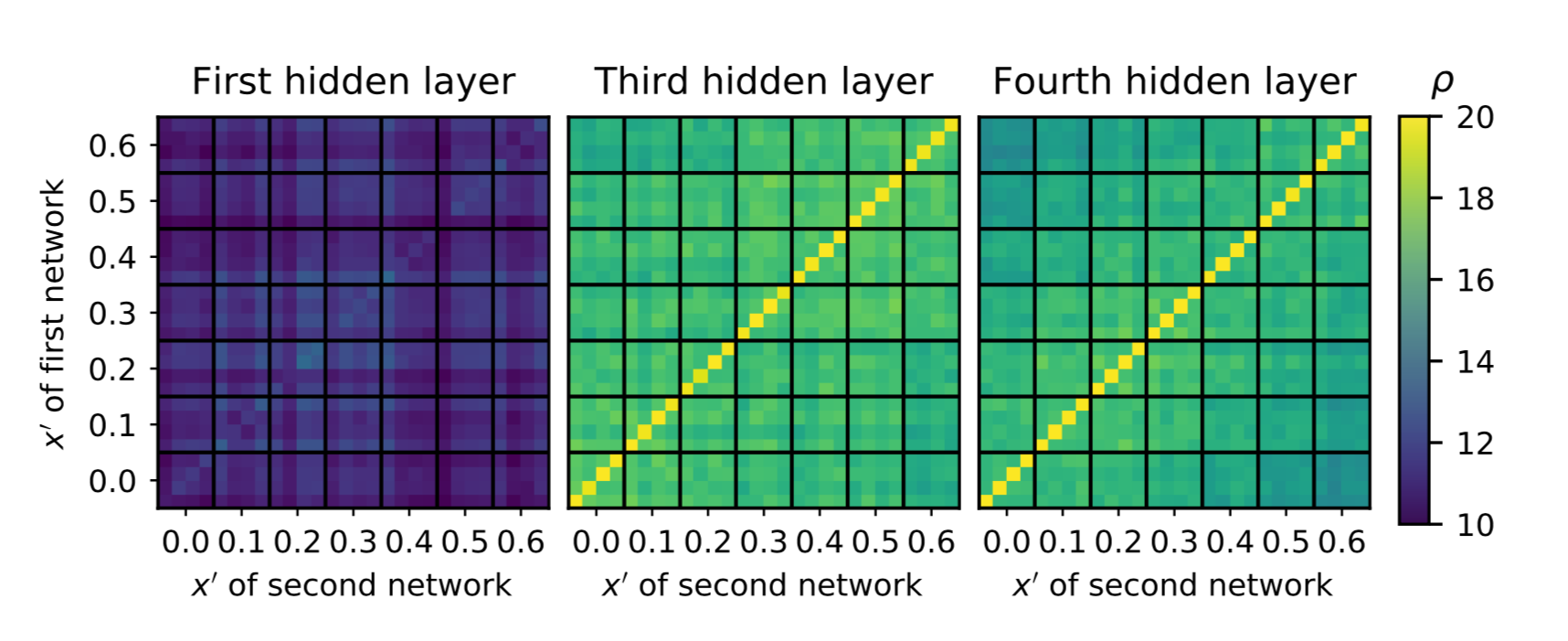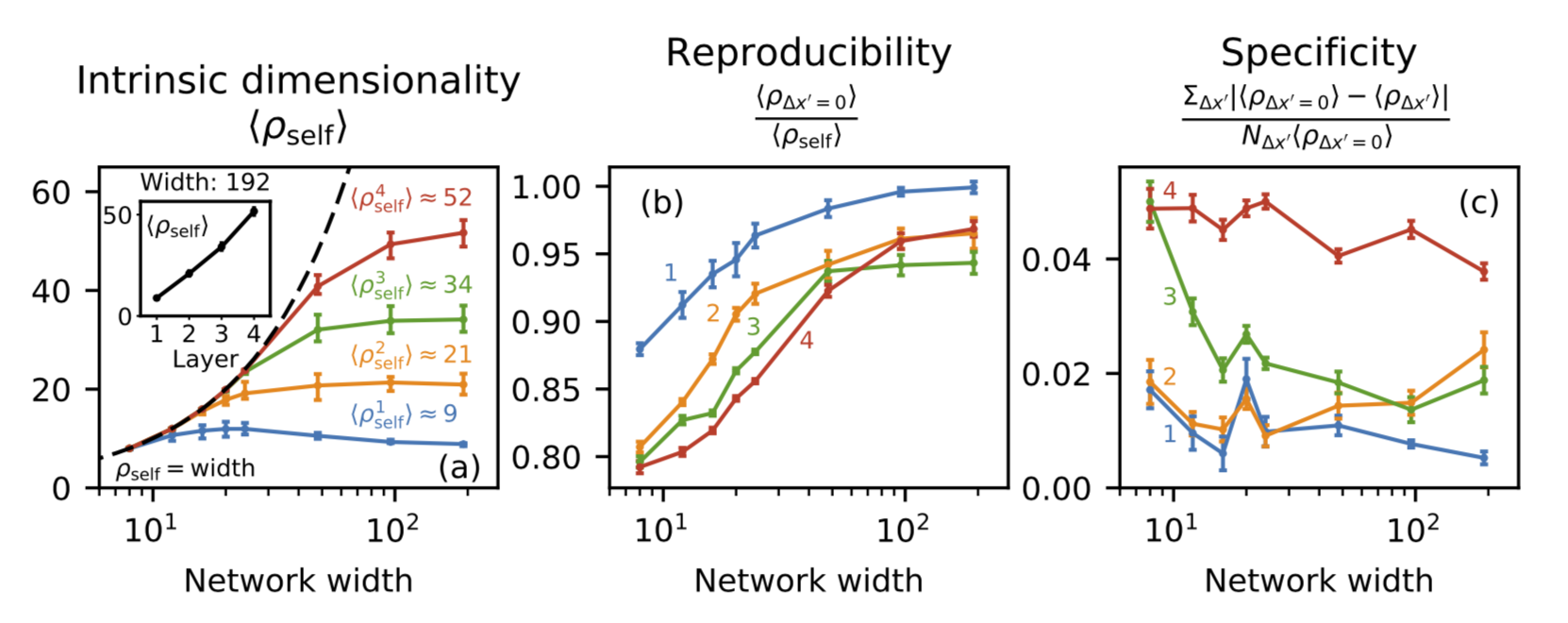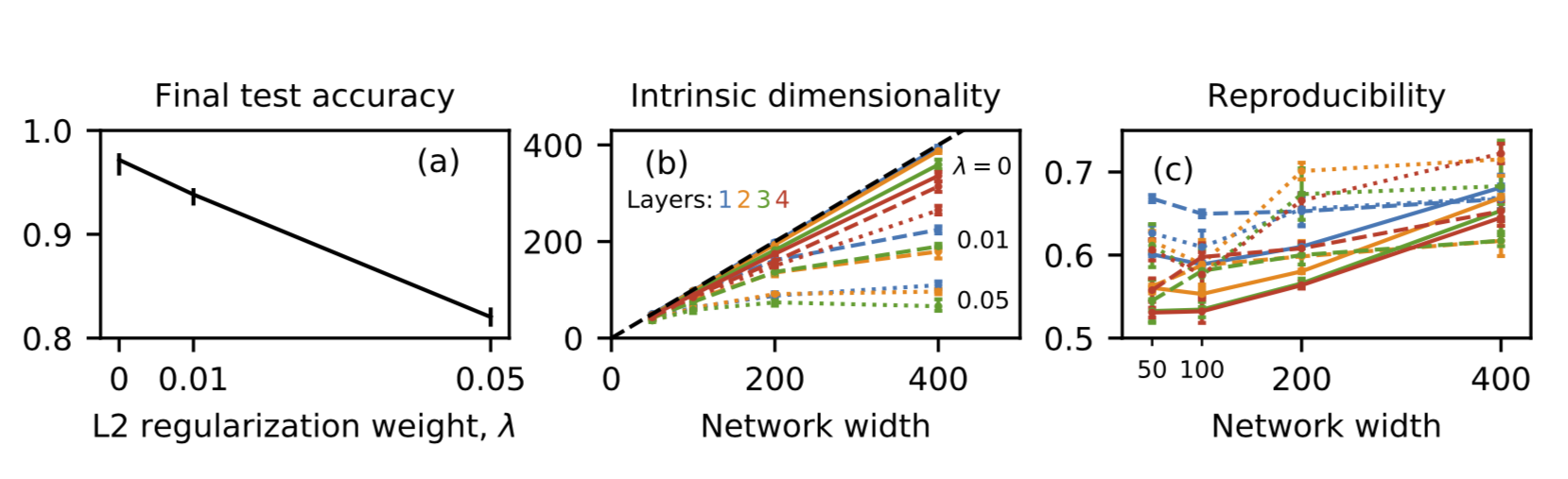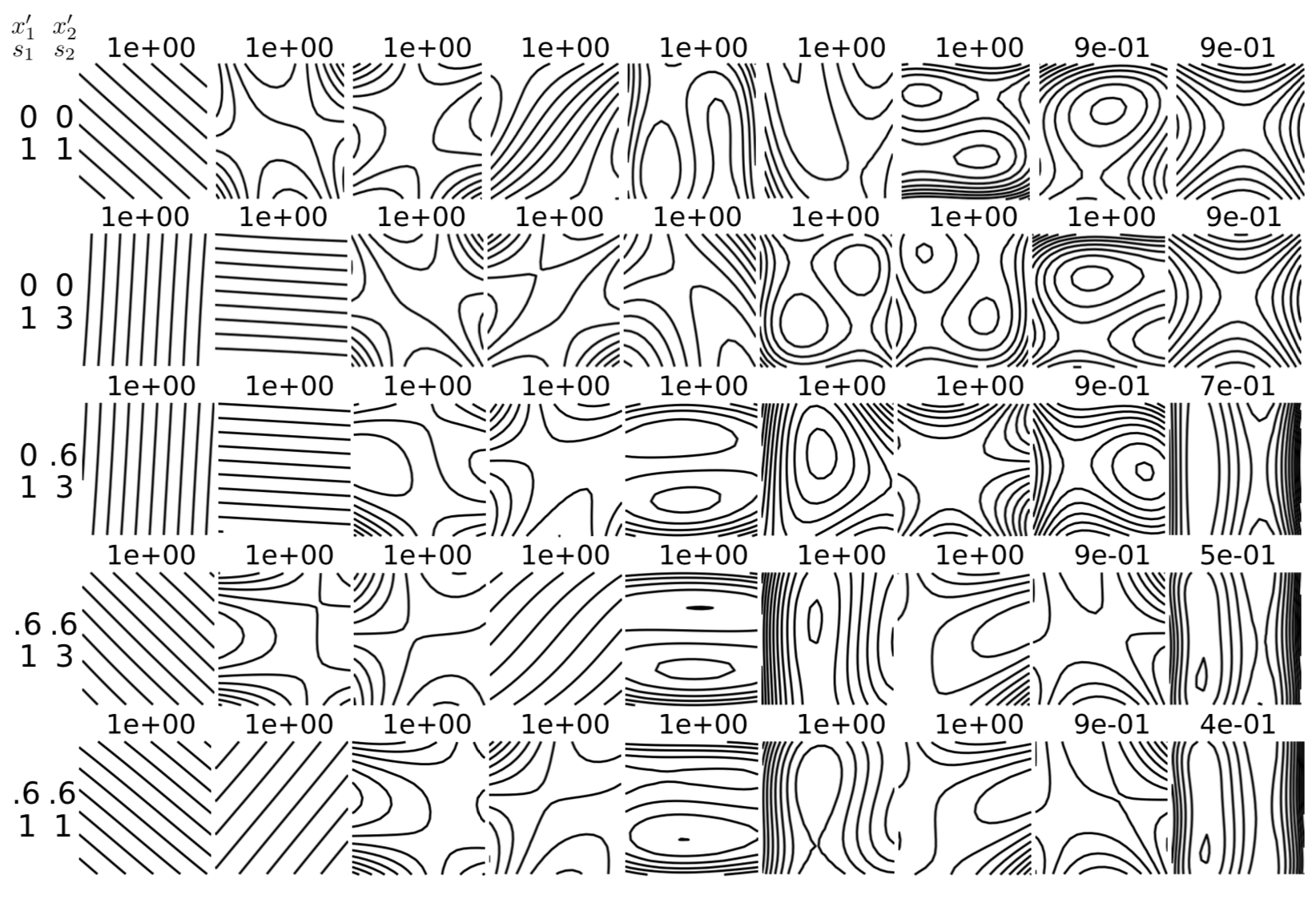# Neural Networks Trained to Solve Differential Equations Learn General Representations

Computational NanoBiophysics Lab1 Visual Computing Lab2
Faculty of Science
University of Ontario Institute of Technology
2000 Simcoe St. N., Oshawa ON L1G 0C5

# Introduction

This work studies the generality of layers across a continuously-parametrized set of tasks: a group of similar problems whose details are changed by varying a real number. We found the transfer learning method for measuring generality prohibitively expensive for this task. Instead, by relating generality to similarity, we develop a computationally efficient measure of generality that uses the singular vector canonical correlation analysis (SVCCA). We demonstrate our method by measuring layer generality in neural networks trained to solve differential equations.

## Layer generality in DENNs using SVCCAMatrices of layer-wise SVCCA similarities between the first, third, and fourth hidden layers of networks of width 20 trained at various x' values, with four random seeds per position. The black lines group layers on each axis by the x' values at which they were trained. For each x' value, the four entries correspond to four distinct random seeds. Thus the matrix diagonals contain self-similarities, the block diagonals formed by black lines contain similarities across random seeds at a fixed x', and the remaining entries correspond to comparisons between distinct x' values.

## Intrinsic dimensionality, reproducibility, and specificityThe intrinsic dimensionality, reproducibility, and specificity of the four layers at varying width. The lines indicate mean values. The error bars on intrinsic dimensionality indicate maxima and minima, whereas the error bars on reproducibility and specificity indicate estimated uncertainty on the means (discussed in the supplemental material). Numbers indicate layer numbers. The inset in (a) shows the limiting dimensionalities of the four layers at width 192.

### MNIST DatasetTest accuracies, intrinsic dimensionalities and reproducibilities of networks trained on the MNIST dataset for various L2 regularization weights $$\lambda$$ and network widths. The error bars in (a) and (b) show maxima and minima; those in (c) show the estimated standard error.

#### Canonical componentsEach row shows plots of the first nine canonical components found by applying the SVCCA between the first layer of a network trained on x'=x'_1 and the first layer of a network trained on a different random seed at x'=x'_2, as indexed to the left of the plots. The number above each plot shows the correlation between the layers in the direction corresponding to that component.

# Posters

## NeurIPS 2018 Poster

Full resolution version is avaialable here.

## NeurIPS 2018 Workshop on Compact Deep Neural Networks with Industrial Applications Poster

Full resolution version is avaialable here.